Parenting
30 Fun Maths Questions with Answers
1.7m views

 1 Introduction 2 30 Fun Maths Questions with answers 3 Conclusion 4 About Cuemath

## Introduction

Mathematics can be fun if you treat it the right way. Maths is nothing less than a game, a game that polishes your intelligence and boosts your concentration. Compared to older times, people have a better and friendly approach to mathematics which makes it more appealing. The golden rule is to know that maths is a mindful activity rather than a task.

There is nothing like hard math problems or tricky maths questions, it’s just that you haven’t explored mathematics well enough to comprehend its easiness and relatability. Maths tricky questions and answers can be transformed into fun math problems if you look at it as if it is a brainstorming session. With the right attitude and friends and teachers, doing math can be most entertaining and delightful.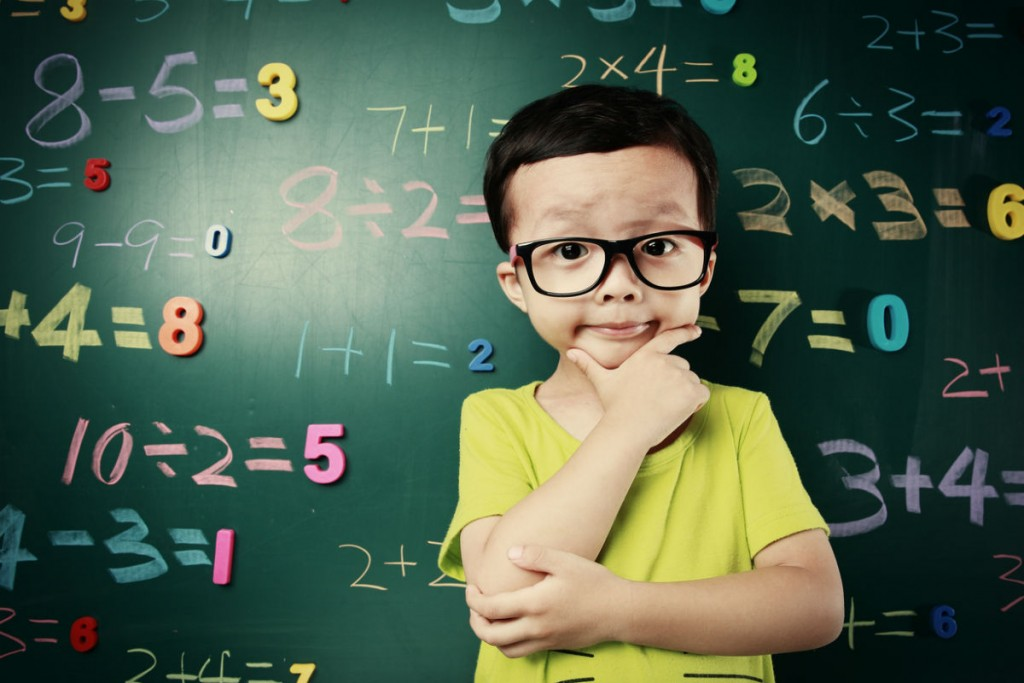Math is interesting because a few equations and diagrams can communicate volumes of information. Treat math as a language, while moving to rigorous proof and using logical reason for performing a particular step in a proof or derivation.

Treating maths as a language totally eradicates the concept of hard math problems or tricky maths questions from your mind. Introducing children to fun maths questions can create a strong love and appreciation for maths at an early age. This way you are setting up the child’s successful future. Fun math problems will urge your child to choose to solve it over playing bingo or baking.

Apparently, there are innumerable methods to make easy maths tricky questions and answers. This includes the inception of the ideology that maths is simpler than their fear. This can be done by connecting maths with everyday life. Practising maths with the aid of dice, cards, puzzles and tables reassures that your child effectively approaches Maths.

If you wish to add some fun and excitement into educational activities, also check out

Cuemath is one of the world's leading math learning platforms that offers LIVE 1-to-1 online math classes for grades K-12. Our mission is to transform the way children learn math, to help them excel in school and competitive exams. Our expert tutors conduct 2 or more live classes per week, at a pace that matches the child's learning needs.

## Fun Maths Questions with answers - PDF

Here are some fun, tricky and hard to solve maths problems that will challenge your thinking ability.

1. If 1=3

2=3

3=5

4=4

5=4

Then, 6=?

Answer: is 3, because ‘six’ has three letters

 3

1. What is the number of parking space covered by the car?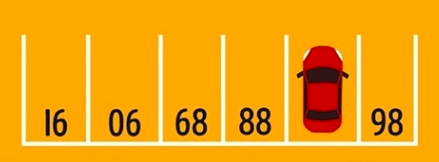This tricky math problem went viral a few years back after it appeared on an entrance exam in Hong Kong… for six-year-olds. Supposedly the students had just 20 seconds to solve the problem!

 87

Believe it or not, this “math” question actually requires no math whatsoever. If you flip the image upside down, you’ll see that what you’re dealing with is a simple number sequence.

1. Replace the question mark in the above problem with the appropriate number.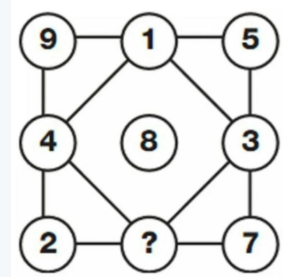6

1. Which number is equivalent to 3^(4)÷3^(2)

This problem comes straight from a standardized test given in New York in 2014.

 9

1. There are 49 dogs signed up for a dog show. There are 36 more small dogs than large dogs. How many small dogs have signed up to compete?

This question comes directly from a second grader's math homework.

 42.5

To figure out how many small dogs are competing, you have to subtract 36 from 49 and then divide that answer, 13 by 2, to get 6.5 dogs, or the number of big dogs competing. But you’re not done yet! You then have to add 6.5 to 36 to get the number of small dogs competing, which is 42.5. Of course, it’s not actually possible for half a dog to compete in a dog show, but for the sake of this math problem let’s assume that it is.

 13.3922

Adding two decimals together is easier than it looks. Don’t let the fact that 8.563 has fewer numbers than 4.8292 trip you up. All you have to do is add a 0 to the end of 8.563 and then add like you normally would.

1. I am an odd number. Take away one letter and I become even. What number am I?

Seven (take away the ‘s’ and it becomes ‘even’).

 Seven

1. Using only an addition, how do you add eight 8’s and get the number 1000?

888 + 88 + 8 + 8 + 8 = 1000

 1000

1. Sally is 54 years old and her mother is 80, how many years ago was Sally’s mother times her age?

41 years ago, when Sally was 13 and her mother was 39.

 41 years ago

1. Which 3 numbers have the same answer whether they’re added or multiplied together?

 1, 2 and 3

1. There is a basket containing 5 apples, how do you divide the apples among 5 children so that each child has 1 apple while 1 apple remains in the basket?

4 children get 1 apple each while the fifth child gets the basket with the remaining apple still in it.

1. There is a three-digit number. The second digit is four times as big as the third digit, while the first digit is three less than the second digit. What is the number?

 141

1. Fill in the question mark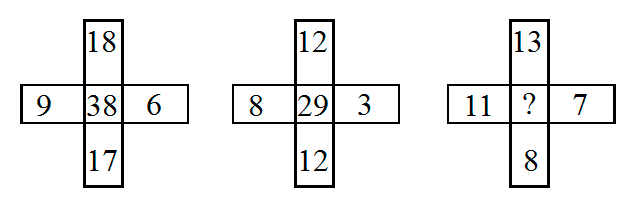25

1. Two girls were born to the same mother, at the same time, on the same day, in the same month and the same year and yet somehow they’re not twins. Why not?

Because there was a third girl, which makes them triplets!

 Triplets

1. A ship anchored in a port has a ladder which hangs over the side. The length of the ladder is 200cm, the distance between each rung in 20cm and the bottom rung touches the water. The tide rises at a rate of 10cm an hour. When will the water reach the fifth rung?

The tide raises both the water and the boat so the water will never reach the fifth rung.

 Never

1. The day before yesterday I was 25. The next year I will be 28. This is true only one day in a year. What day is my Birthday?

 December 31

1. You have a 3-litre bottle and a 5-litre bottle. How can you measure 4 litres of water by using 3L and 5L bottles?

Solution 1 :

• First, fill 3Lt bottle and pour 3 litres into 5Lt bottle.

• Again fill the 3Lt bottle. Now pour 2 litres into the 5Lt bottle until it becomes full.

• Now empty 5Lt bottle.

• Pour remaining 1 litre in 3Lt bottle into 5Lt bottle.

• Now again fill 3Lt bottle and pour 3 litres into 5Lt bottle.

• Now you have 4 litres in the 5Lt bottle. That’s it.

Solution 2 :

• First, fill the 5Lt bottle and pour 3 litres into 3Lt bottle.

• Empty 3Lt bottle.

• Pour remaining 2 litres in  5Lt bottle into 3Lt bottle.

• Again fill the 5Lt bottle and pour 1 litre into 3 Lt bottle until it becomes full.

• Now you have 4 litres in the 5Lt bottle. That’s it.

1. 3 Friends went to a shop and purchased 3 toys. Each person paid Rs.10 which is the cost of one toy. So, they paid Rs.30 i.e. total amount. The shop owner gave a discount of Rs.5 on the total purchase of 3 toys for Rs.30. Then, among Rs.5, Each person has taken Rs.1 and remaining Rs.2 given to the beggar beside the shop. Now, the effective amount paid by each person is Rs.9 and the amount given to the beggar is Rs.2. So, the total effective amount paid is 9*3 = 27 and the amount given to beggar is Rs.2, thus the total is Rs.29. Where has the other Rs.1 gone from the original Rs.30?

The logic is payments should be equal to receipts. We cannot add the amount paid by persons and the amount given to the beggar and compare it to Rs.30.The total amount paid is ₹27. So, from ₹27, the shop owner received 25 rupees and beggar received ₹ 2. Thus, payments are equal to receipts.

1. How to get a number 100 by using four sevens (7’s) and a one (1)?

Answer 1: 177 – 77 = 100 ;

Answer 2: (7+7) * (7 + (1/7)) = 100

 100

1. Move any four matches to get 3 equilateral triangles only (don’t remove matches)Move 2, 4, 5, 6 matches

1. Find the area of the red triangle.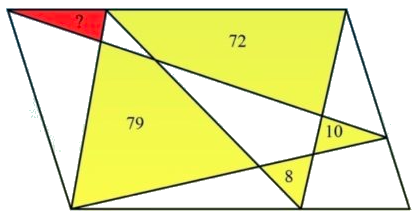9

To solve this fun maths question, you need to understand how the area of a parallelogram works. If you already know how the area of a parallelogram and the area of a triangle are related, then adding 79 and 10 and subsequently subtracting 72 and 8 to get 9 should make sense.

1.  How many feet are in a mile?

 5280

1. Solve  - 15+ (-5x) =0

 -3

1. What is 1.92÷3

 0.64

1. A man is climbing up a mountain which is inclined. He has to travel 100 km to reach the top of the mountain. Every day He climbs up 2 km forward in the day time. Exhausted, he then takes rest there at night time. At night, while he is asleep, he slips down 1 km backwards because the mountain is inclined. Then how many days does it take him to reach the mountain top?

 99 Days

1.  If 72 x 96 = 6927, 58 x 87 = 7885, then 79 x 86 = ?

 6897

1. Look at this series: 36, 34, 30, 28, 24, … What number should come next?

 22

1.  Look at this series: 22, 21, 23, 22, 24, 23, … What number should come next?

 25

1. If 13 x 12 = 651 & 41 x 23 = 448, then, 24 x 22 =?

 924

1. Look at this series: 53, 53, 40, 40, 27, 27, … What number should come next?

 14

## Conclusion

The ultimate goals of mathematics instruction are students understanding the material presented, applying the skills, and recalling the concepts in the future. There's little benefit in students recalling a formula or procedure to prepare for an assessment tomorrow only to forget the core concept by next week.

Teachers must focus on making sure that the students understand the material and not just memorize the procedures. After you learn the answers to a fun maths question, you begin to ask yourself how you could have missed something so easy. The truth is, most trick questions are designed to trick your mind, which is why the answers to fun maths questions are logical and easy.